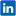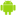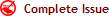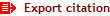|Twitter |Facebook |Google+ |VKontakte | LinkedIn|Viadeo | English | Français | Español | العربية |International Journal of Innovation and Applied Studies
ISSN: 2028-9324     CODEN: IJIABO     OCLC Number: 828807274     ZDB-ID: 2703985-7
 Tuesday 09 August 2022

# Connect with IJIAS

••••••••Now IJIAS is indexed in EBSCO, ResearchGate, ProQuest, Chemical Abstracts Service, Index Copernicus, IET Inspec Direct, Ulrichs Web, Google Scholar, CAS Abstracts, J-Gate, UDL Library, CiteSeerX, WorldCat, Scirus, Research Bible and getCited, etc.

# Mathematical Expressions for Estimation of Errors in the Formulas which are used to obtaining intermediate values of Biological Activity in QSAR

Volume 2, Issue 3, March 2013, Pages 272–279Nizam Uddin1

Original language: English

Copyright © 2013 ISSR Journals. This is an open access article distributed under the Creative Commons Attribution License, which permits unrestricted use, distribution, and reproduction in any medium, provided the original work is properly cited.

# Abstract

Quantitative structure-activity relationships (QSAR) attempts to find consistent relationships between the variations in the values of molecular properties and the biological activity for a series of compounds. These physicochemical descriptors, which include parameters to account for hydrophobicity, topology, electronic properties, and steric effects, are determined empirically or, more recently, by computational methods. Quantitative structure-activity relationships (QSAR) generally take the form of a linear equation where the biological activity is dependent variable. Biological activity is depended on the parameters and the coefficients. Parameters are computed for each molecule in the series. Coefficients are calculated by fitting variations in the parameters. Intermediate values of the biological activity are obtained by some formulas. These formulas are worked in tabulated values of biological activity in Quantitative structure-activity relationships. These formulas are worked in the conditions and all conditions are based on the position of the point lies in the table. Derived formulas using Newton's method for interpolation are worked in conditions which are depending on the point lies. If the point lies in the upper half then used Newton's forward interpolation formula. If the point lies in the lower half then we used Newton's backward interpolation formula. And when the interval is not equally spaced then used Newton's divide difference interpolation formula. When the tabulated values of the function are not equidistant then used Lagrangian polynomial. Mathematical expressions are derived for estimation of errors using intermediate values and formulas.

Author Keywords: Biological activity, Estimation of Errors, Intermediate values, Mathematical Expressions, QSAR.